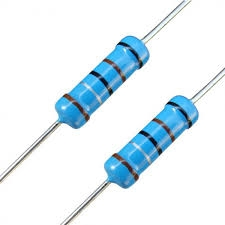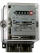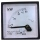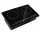# Electric input power

Solve problems related to electric power:

a) U = 120 V, I = 0.5 A, P =?
b) P = 200 W, U = 230 V, I =?
c) I = 5 A, P = 2200W, U =?

P1 =  60 W
I2 =  0.8696 A
U3 =  440 V

### Step-by-step explanation:Did you find an error or inaccuracy? Feel free to write us. Thank you!## Related math problems and questions:

• Electric workCalculate the work done by the electric forces passing the current of 0.2 A through the bulb in 10 minutes if the bulb is connected to a 230 V power supply.
• ResistanceDetermine the resistance of the bulb with the current 200 mA and is in a regular lamp (230V).
• Mains voltageHow much electric current flows through the appliance with a resistance of 40kΩ, which is connected to the mains voltage (230 V)?
• Filament of bulbThe filament of bulb has a 1 ohm resistivity and is connected to a voltage 220 V. How much electric charge will pass through the fiber when the electric current passes for 10 seconds?
• Energy consumptionThe device is connected to 230V and draws 3.5A current. Power consumption is 1932kJ. How many minutes has this device been in operation?
• TransformerTransformer - U1 = 230 V, N1 = 300, N2 = 1,200, I1 = 4 A. Calculate the transformation ratio, voltage and current in the secondary coil.
• CookerA current of 2A passes through the immersion cooker at a voltage of 230V. What work do the electric field forces in 2 minutes?
• TransformerSolve the textbook problems - transformer: a) N1 = 40, N2 = 80, U2 = 80 V, U1 =? b) N1 = 400, U1 = 200 V, U2 = 50 V, N2 =?
• The bulbWhat is the resistance of a filament lamp when it is connected to a 9V battery and has a current of 120 mA?
• Kirchhoff's lawTwo resistors with 100 Ω and 300 Ω resistors are connected in series. A current of 1.8 A passes through the first 100Ω resistor. What current flows through the second resistor?
• Circuit voltageCalculate the closed-circuit voltage. Calculate the power on the resistors. R1 = 30 ohms, R2 = 10 ohms. I = 0.1A. U =?. The resistors are connected in series, one after the other.
• Two resistorsTwo resistors 20 Ω and 60 Ω are connected in series and an external voltage of 400 V is connected to them. What are the electrical voltages on the respective resistors? Please comment!
• Coils of transformerThe primary coil of the transformer has 400 turns, a current of 1.5 A passes through it and is connected to a voltage of 220 V. For the secondary coil, find the voltage, current, and a number of turns if the transformation ratio k = 0.1.
• Closed circuitIn a closed circuit, there is a voltage source with U1 = 12 V and with an internal resistance R1 = 0.2 Ω. The external resistance is R2 = 19.8 Ω. Determine the electric current and terminal voltage.
• Electric cookerDuring which time t does an electric cooker with power input P = 500 W and with efficiency n = 75% heat water with mass m = 2 kg and temperature t1 = 10°C to the boiling point (t2 = 100°C). The specific heat capacity of water is c = 4 180 J. Kg-1. K-1
• ResistanceA resistor having an electrical resistance of 1.5 k ohms passes an electrical current of 0.1 A. Calculate what voltage is between the terminals of the resistor.
• Current in the conductorCalculate the current in the conductor (in mA) if it is connected to a 4.5 V voltage source and its resistance is 20 (ohm).